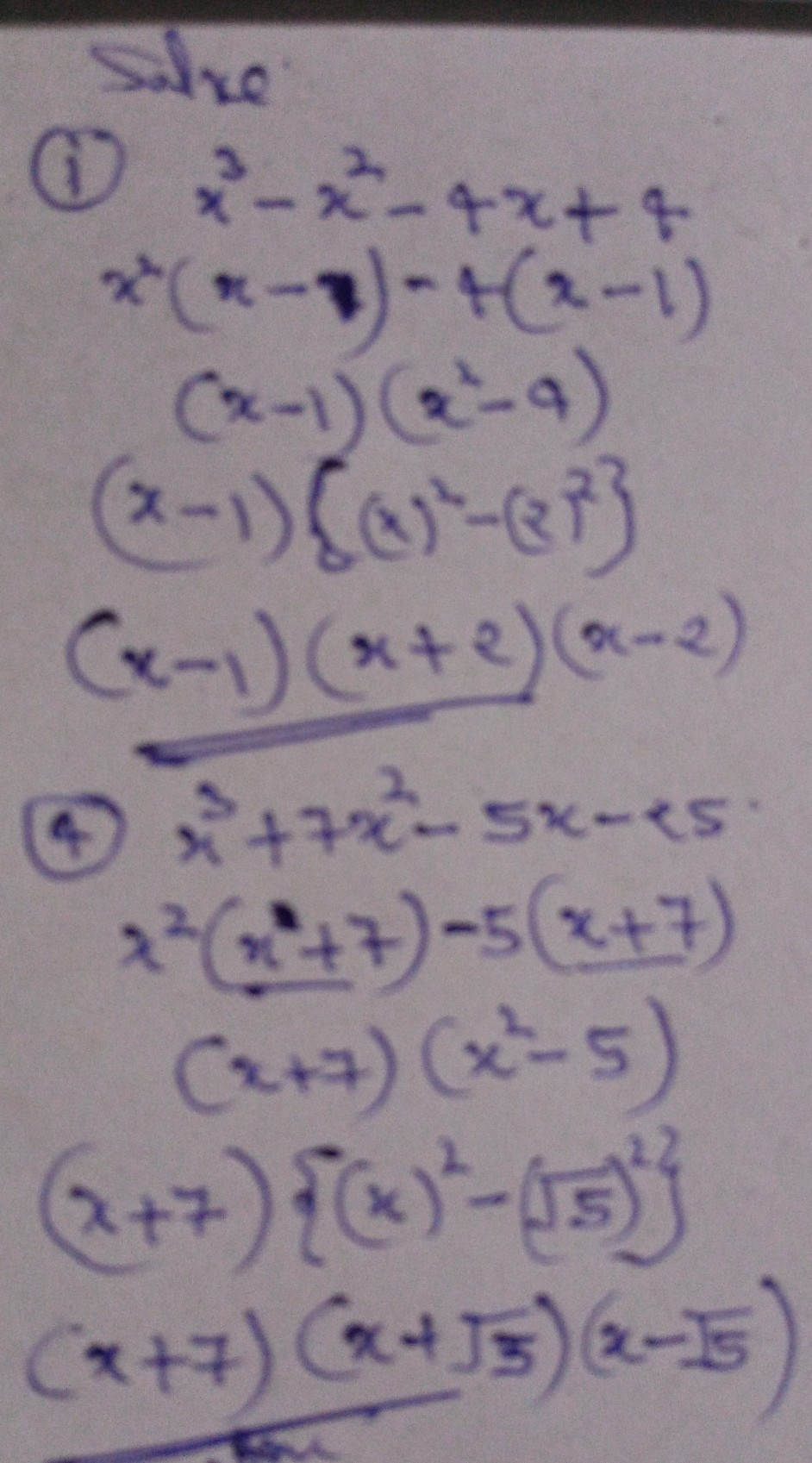ProblemSolutionQanda teacher - SUDESHStudent

where's the number 12345?

you answer only 1 and 4 where's the 2,3,and 5

Similar problem